# Random Number Generation Part II – Some Data Analysis

Following on from my last post, I’ve been trying to read the voltage values that are generated from the random number generator I put together. I was initially using an arduino unit so I could control the voltage output down to below 1 volt. Ultimately I want to read the values with an ESP8266 unit running micropython so I can upload the values to an internet based data logger and the ESP8266 is only 1 volt tolerant on its analog-to-digital pin. So, the voltage has to be controlled and I didn’t mind blowing up one of my arduinos (which are 5 volt tolerant). The voltage divider is now composed of a 10k resistor from positive rail to analog out and a 1k resistor from analog out to ground.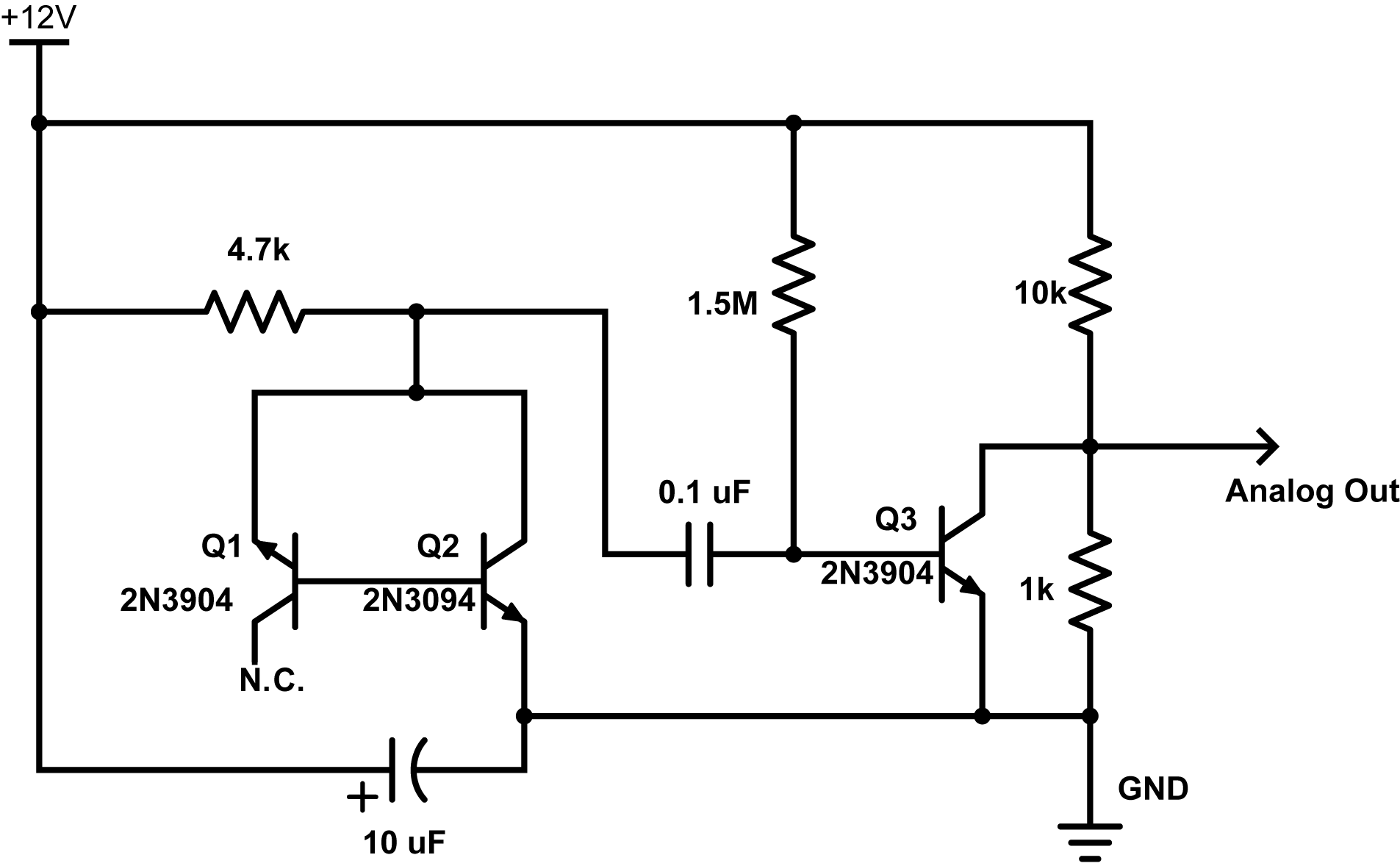The ardunio was giving me readings up to about 180 unit out of 1024 which gives about 180/1024 * 5V = 0.8789 volts… nice and safe.

Reading these voltages with the ESP8266 gave some interesting results. The code to do this was very simple:

```from machine import ADC

import time

for i in range(1000000):
time.sleep_ms(10)```

This reads a voltage every 10 milliseconds from the analog pin and reports one million of them. It took about 2.8 hours and the output was redirected to a file of type csv with this command on my mac:

`ampy --port /dev/tty.SLAB_USBtoUART run readanalog.py > avalanche_noise.csv`

‘ampy’ is a nice program from Adafruit, which you can learn more about here.

I then analyzed this data in python using the jupyter web interface. A histogram plot of the values looks like this: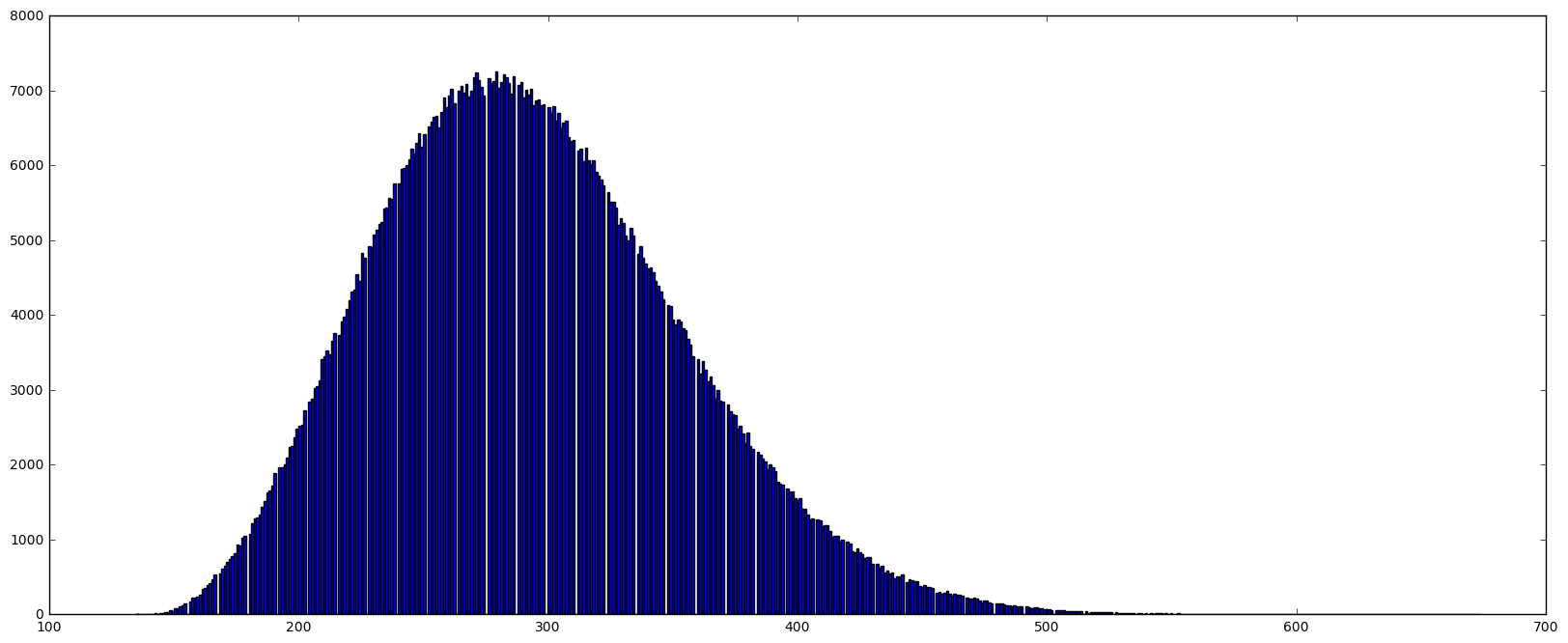The values range from 135 to 674 with a mean of ~292.6. This distribution is obviously skewed too so it’s not gaussian distributed – maybe Poisson?  But the most striking thing is the lines of empty space (no blue) – is this a plotting problem or are certain values skipped during the reads? Well lets zoom in around the top of the distribution.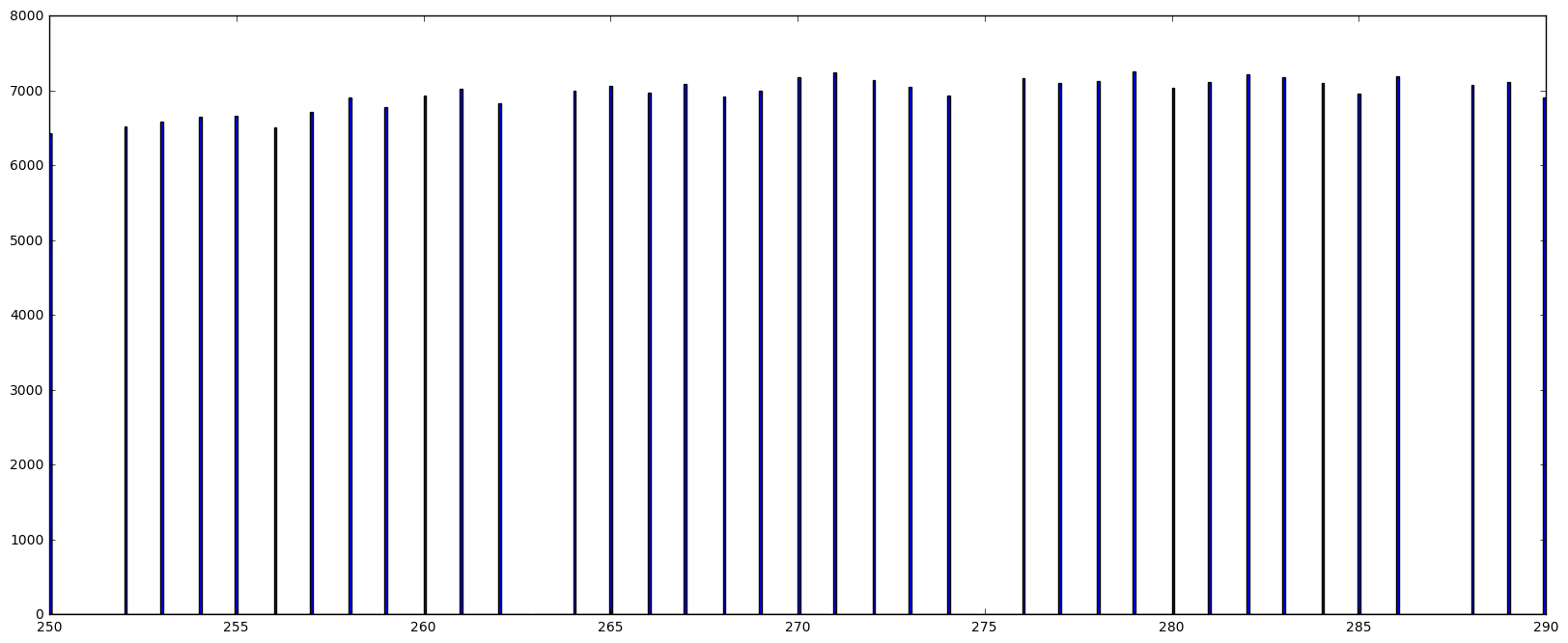So its true, some values are just never recorded. Its hard to believe the voltages from the circuit would do this. Also, its clear from the numbers that every 12th value is skipped. I think this must be an error in the way micropython reads the register so I’ll probably post this somewhere in the micropython forums and see if this is true or if I’m just doing something wrong.

Next I tried to fit this distribution to a gaussian curve. It failed. Here is the python code:

```import math
import numpy as np
import matplotlib.pyplot as plt
import scipy as scipy
import scipy.stats as stats
from scipy.optimize import curve_fit
from scipy.misc import factorial
from scipy.stats import norm
%matplotlib inline          # needed for plotting in jupyter

data_p = data # not really needed

fig = plt.figure(figsize=(20, 8))
entries, bin_edges, patches = plt.hist(data_p, bins=(int(np.amax(data_p)-np.amin(data_p))), range=[np.amin(data_p),np.amax(data_p)], normed=True)
bin_middles = 0.5*(bin_edges[1:] + bin_edges[:-1])

# poisson function, parameter lamb is the fit parameter
def gauss(x, *p):
A, mu, sigma = p
return A*np.exp(-(x-mu)**2/(2.*sigma**2))

# fit with curve_fit
p0 = [1., 260., 30.] # initial guesses for height, mean and SD

parameters, cov_matrix = curve_fit(gauss, bin_middles, entries, p0 = p0)
print parameters # print results
print np.sqrt(np.diag(cov_matrix)) # print errors of fit

# plot poisson-deviation with fitted parameter
x_plot = np.linspace(0, np.amax(data_p), 1000)
plt.plot(x_plot, gauss(x_plot, *parameters), 'r-', lw=4)
plt.show()```

Output: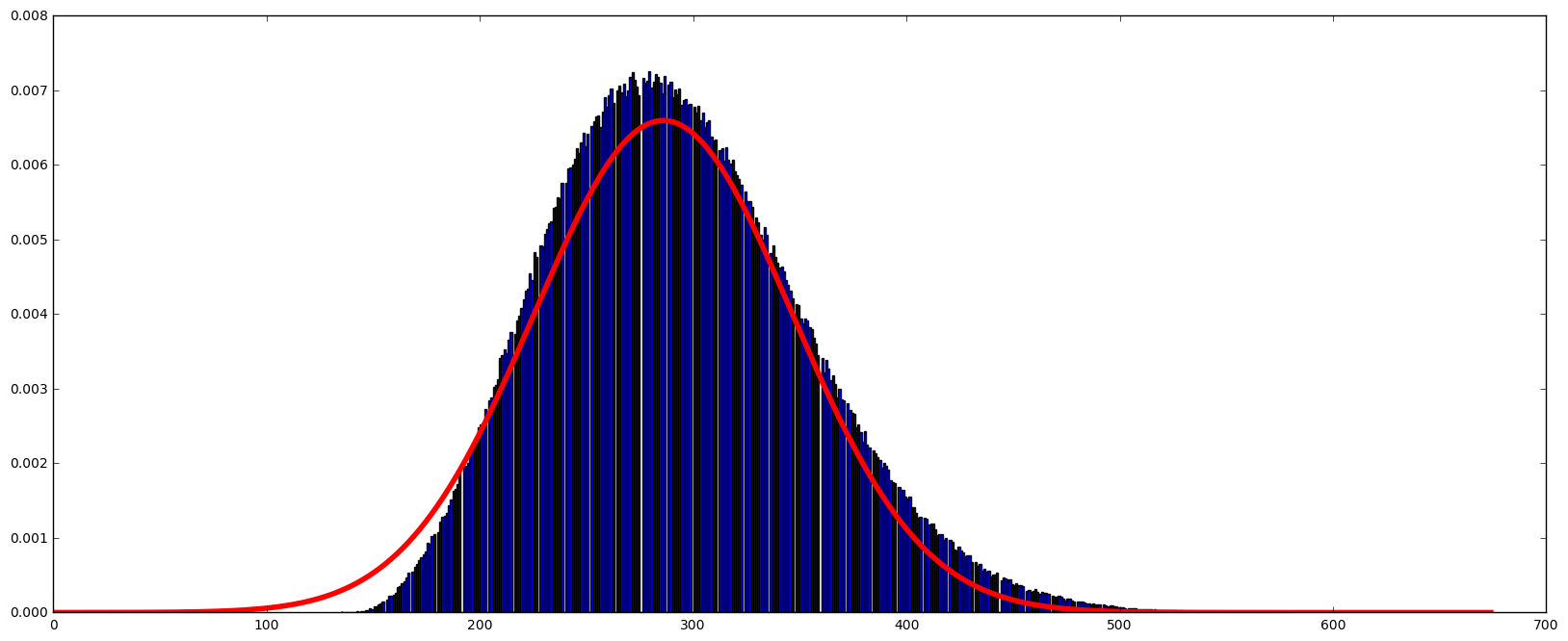This doesn’t fit very well. But it does reveal the skew present in the data. It does in some ways look poisson distributed (you can read more about this distribution here) and electrical noise is typically poisson distributed because of the discrete nature of electrical charge (read about this and shot noise here).

Mathematical aside:

One way I like to think of the Poisson distribution and Poisson processes is as follows. They arise from discrete events that must always have a positive value – this is not true for Gaussian distributions. So, in our circuit, current (electrons) flow or do not flow. When they flow, a discrete (integer) number of them flow. There is a lower limit on the number that can jump the gap. That number is zero. The gaussian distribution is the limit of a binomial distribution as the number of events goes to infinity. The binomial distribution is based on the idea that an event either happens or does not. (N.B. this is different from the Poisson case because in that case, events either happen 1 or more times or do not happen). If we accumulate binomial events, there is no limit to how many ‘yes’ or ‘no’ events can happen so some of the distribution must extend as far as the number of events recorded – for gaussian this limit goes to infinity and negative infinity. The Poisson distribution doesn’t act this way. If we try and model the Poisson distribution as an infinite binomial distribution we quickly realize that while we can get an infinite number of ‘zero value’ events as well (with low probability) there is more than one other alternative. So the distribution must take into account these many possible values which stretches the distribution in the positive direction while there is a still a hard limit at zero. We can shift the Poisson distribution so ‘N’ zero-value trials will be plotted at ‘-N’ (like we would for a binomial distribution) but on the positive size, the curve would extend past ‘+N’ because some of those trials can have a value of more than 1.

Enough qualitative description of distributions…

Using a poisson function instead like this:

```def poisson(k, lamb):
return (lamb**k/factorial(k)) * np.exp(-lamb)```

and we get this: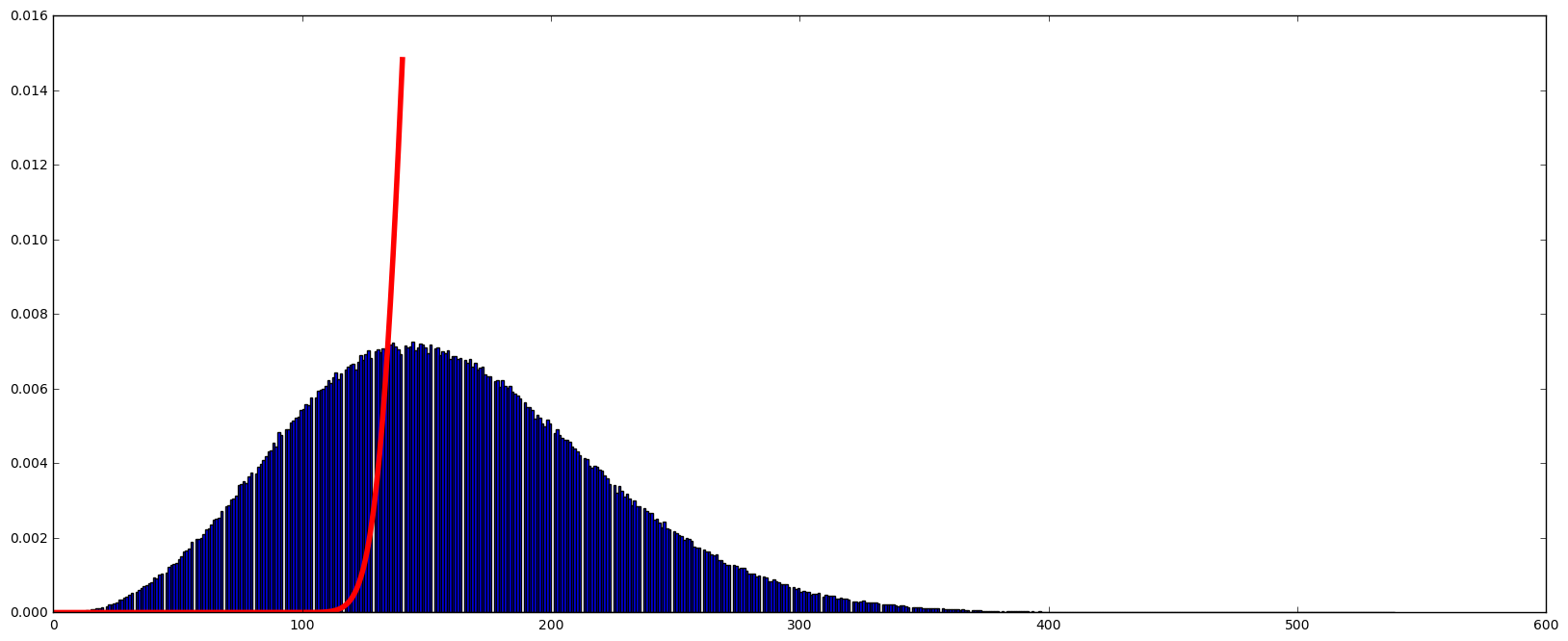It doesn’t converge at all.

Yikes, whats going on?

Well my thought was that the numbers I’m reading don’t match the number of electrons actually flowing. This signal is amplified by the transistors and then quantized, not in nature, but by the ADC converter in the ESP8266. My hope is that this signal is proportional to the number of electrons that flowed during signal acquisition. But this signal is not the same as the number of electrons which will be behaving as a Poisson process. But the recorded numbers should be proportional to the number of electrons. So if we divide these values by some constant, can we get the fit to work, and at what optimal division factor.

Long story short, if I divide the 1000000 million points by 23.9 I get an optimal fit in terms of the error reported for the fitted parameter. That parameter, which is the mean value, is ~6.558336. Does this mean, that on average 6.5 electrons pass through the transistor while the ESP8266 is taking an ADC measurement? I think it might be! Here is the fit: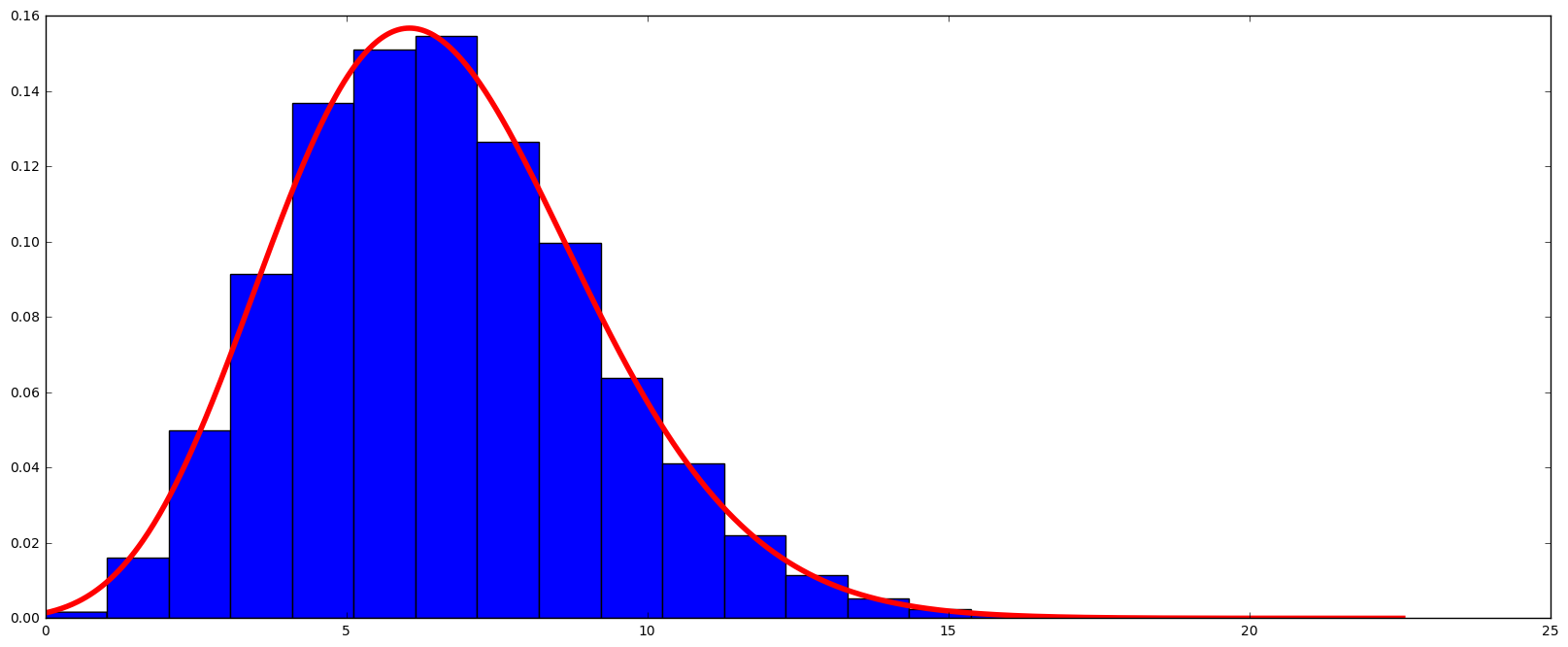If I take these same numbers and try and fit a Gaussian curve, it doesn’t do as well.Conclusions? The electrons that flow across the junction in reverse bias are behaving as a Poisson process as expected. The distribution is not flat. I’ve seen some discussion on the net where people seem to assume this would be the case. It does seem to be random! One of the next steps is to convert these numbers to a flat distribution or at least make it generate a binary sequence. It seems to be that XORing  or Von Neumann filtering will not do a good job of removing the biasing that the Poisson distribution will introduce.

This site uses Akismet to reduce spam. Learn how your comment data is processed.